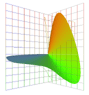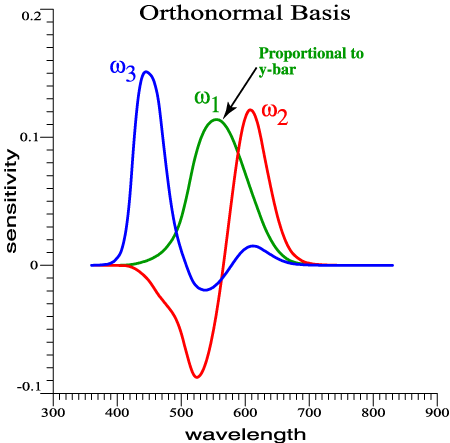Jim Worthey • Lighting & Color Research • jim@jimworthey.com • 301-977-3551 • 11 Rye Court, Gaithersburg, MD 20878-1901, USA

 Vectorial Sensitivity to Wavelength, Now in 3 Dimensions.A set of 3 orthonormal color matching functions. Combining the orthonormal functions into a parametric plot yields Jozef Cohen's "Locus of Unit Monochromats," the eye's Vectorial Sensitivity to Wavelength. Click the image above to see the virtual reality picture.

 Features of the Orthonormal System
1. Axes have intuitive meanings: Achromatic, Red-Green, and Blue-Yellow.
2. The first two functions, ω1(λ) and ω2(λ), combine red and green cones only.
3. ω1(λ) is a multiple of the familiar y-bar.
4. ω1(λ), ω2(λ) and ω3(λ) combine to give the eye's vectorial sensitivity to color.
5. The functions are easily calculated, or get them from this link.
6. A light's tristimulus vector has the same magnitude as the light's "fundamental metamer."
7. Vector amplitude is non-arbitrary and has the units of the stimulus, such as radiance units. (Further explanation?)
8. We don't usually learn to graph tristimulus vectors, or compute their magnitudes. Even graphs of the vector (X Y Z) would give some insight, but graphs and magnitudes mean more here.
9. Algebraic benefit: orthonormal functions simplify derivations and formulas.
10. Beginning students can be told flatly "This is the eye's vectorial sensitivity to color." Details can follow as needed.